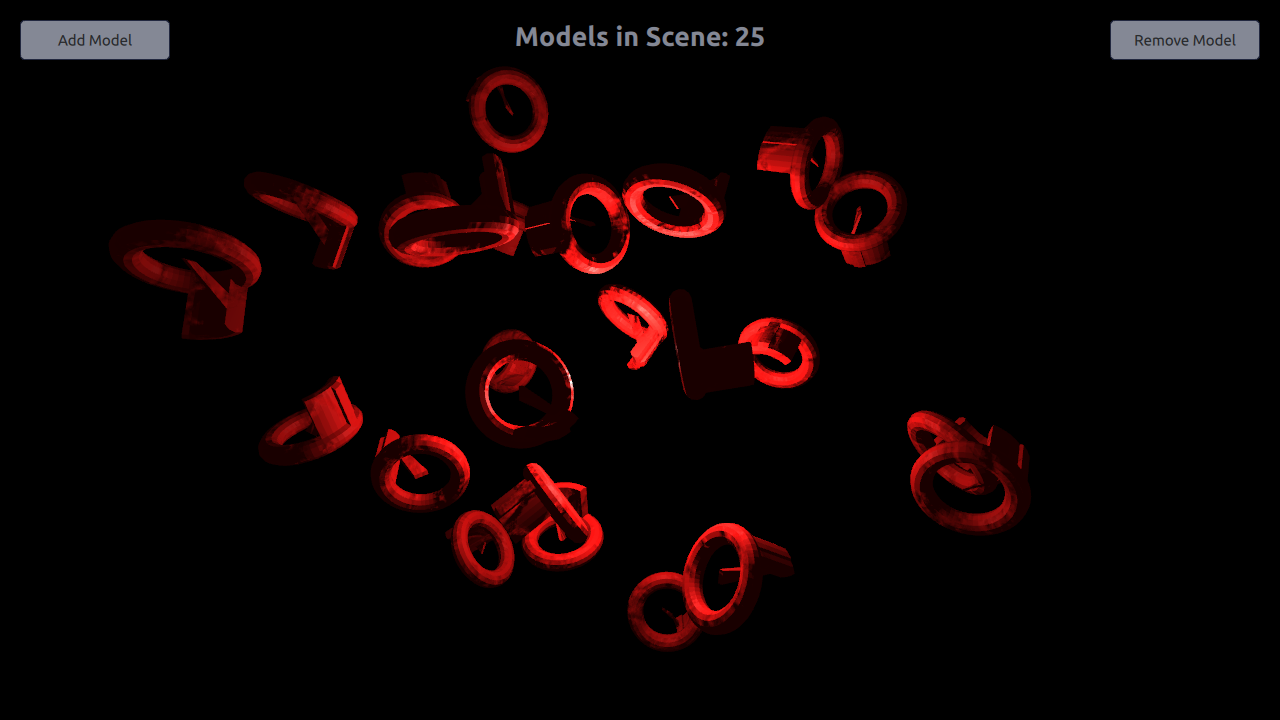# Qt Quick 3D - Dynamic Model Creation Example

Demonstrates dynamic model creation.

This example demonstrates creating models dynamically in an application. 10 models are created dynamically at the start of the application, and more can be added or removed using the `Add Model` and `Remove Model` buttons. Dynamic model count is limited to 100 in this example, but that is in no way a limitation of QtQuick3D.#### Setting Up

##### Spawner Node

We are going to need a Node to hold the dynamically created models.

```Node {
id: shapeSpawner
property real range: 300
property var instances: []

...```
##### Startup

We're creating 10 models at `Component.onCompleted` to avoid opening into an empty view.

```Component.onCompleted: {
// Create 10 instances to get started
for (var i = 0; i < 10; ++i)
}```

#### Dynamic Models

The `addOrRemove()` function called during startup can add or remove the models.

A model component is created using the `Qt.createComponent` function, and instantiated with `position` and `scale` parameters using the `createObject` function.

```// Create a new weirdShape at random postion
var xPos = (2 * Math.random() * range) - range;
var yPos = (2 * Math.random() * range) - range;
var zPos = (2 * Math.random() * range) - range;
var weirdShapeComponent = Qt.createComponent("WeirdShape.qml");
let instance = weirdShapeComponent.createObject(
shapeSpawner, { "x": xPos, "y": yPos, "z": zPos,
"scale": Qt.vector3d(0.25, 0.25, 0.25)});
instances.push(instance);```
##### Removing Models

Dynamically created models are removed simply by popping and destroying them from the instance stack.

```// Remove last item in instances list
let instance = instances.pop();
instance.destroy();```

Files: